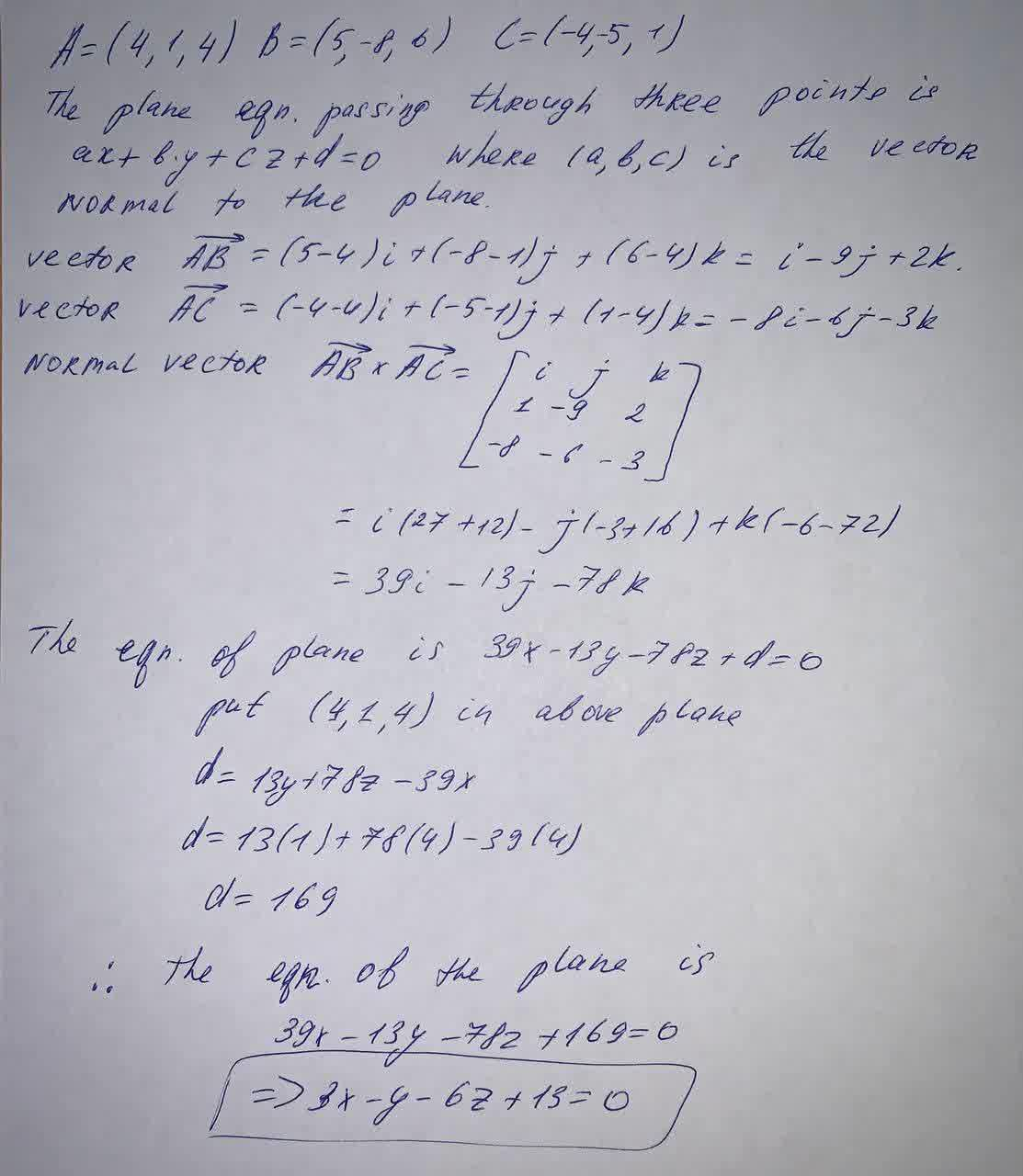Find an equation of the plane.The plane through the points. (4, 1, 4), (5, -8, 6), and (-4, -5, 1)sanuluy 2021-09-25 Answered
Find an equation of the plane.
The plane through the points (4, 1, 4), (5, -8, 6), and (-4, -5, 1)

• Questions are typically answered in as fast as 30 minutes

Solve your problem for the price of one coffee

• Math expert for every subject
• Pay only if we can solve itMaciej Morrow
Solution below